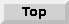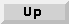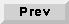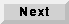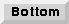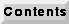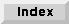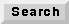## C.2 optfir - equiripple FIR filter design

`Optfir` is a Fortran program performing classical FIR filter design using the Parks-McClellan algorithm. There is nothing unusual about this program, so almost any DSP book will give an adequate explanation of the algorithm as well as the meaning of its arguments. Briefly, the program permits the design of bandpass filters, differentiators, hilbert transformers, and half-band filters.

`Enter name of input command file (press <Enter> for manual entry, Sorry, no tilde-expansion. Give path relative to your home or start-up directory):`
`	<Return> `
You can put your responses in a file to avoid having to type them each time you execute the program. Here, I assume you are typing them interactively.

`Enter filter type (1=Bandpass, 2=Differentiator, 3=Hilbert transformer, 4=Half-band):`
`	1 `
Lowpass, Highpass and Bandpass filters are all called Bandpass.

`Enter filter length (enter 0 for estimate):`
`	32`
The number of taps. If you enter 0, the program figures out how many you need for your specification.

`Enter sampling rate of filter:`
`	1`
Use 1Hz.

`Enter number of filter bands:`
`	2`
There will be a passband and a stopband.

`Enter lower band edge for band 1:`
`	0`
For a lowpass filter, this should be 0 for d.c.

`Enter upper band edge for band 1:`
`	0.1`
Upper edge of the passband.
`Enter desired value for band 1:`
`	1`
Specify unity gain in the passband.

`Enter weight factor for band 1:`
`	1 `
Using 1 here and 1 in the stopband will give ripples of the same size in both bands. You could experiment with allowing more ripple in the passband by making this number smaller.

`Enter lower band edge for band 2:`
`	0.15`
Lower edge of the stopband.

`Enter upper band edge for band 2:`
`	0.5 `
Since this is the Nyquist frequency, it specifies the top of the stopband.

`Enter desired value for band 2:`
`	0`
Zero here defines this to be the stopband.

`Enter weight factor for band 2:`
`	1`
See comment above on weight.

`Do you want x/sin(x) predistortion? (y/n):`
`	n`
Note that the program will design a filter that predistorts to compensate for the effect of the zero-order hold in a D/A converter.

`Enter name of coefficient output file (Sorry, no tilde-expansion. Give path relative to your home directory):`
`	filter_taps`
The name of the file in which to store the impulse response of the design. The resulting file can be used in an FIR star or a WaveForm star using the syntax `< filename` to read it. Note that the file will be stored in your home directory.

` Executing ...`

` Finite Impulse Response (FIR) Linear Phase Digital Filter Design Remez Exchange Algorithm`
` Bandpass Filter Filter length = 32`

` Impulse Response Coefficients:`
` h( 1) = 0.2199929E-02 = h( 32) h( 2) = -0.1615105E-01 = h( 31) h( 3) = -0.1167266E-01 = h( 30) h( 4) = -0.5506404E-02 = h( 29) h( 5) = 0.6444952E-02 = h( 28) h( 6) = 0.1864344E-01 = h( 27) h( 7) = 0.2227415E-01 = h( 26) h( 8) = 0.1105212E-01 = h( 25) h( 9) = -0.1328082E-01 = h( 24) h( 10) = -0.3894535E-01 = h( 23) h( 11) = -0.4806972E-01 = h( 22) h( 12) = -0.2521652E-01 = h( 21) h( 13) = 0.3334328E-01 = h( 20) h( 14) = 0.1151020E+00 = h( 19) h( 15) = 0.1945332E+00 = h( 18) h( 16) = 0.2434263E+00 = h( 17)`

` Lower band edge: 0.0000000 0.1500000 Upper band edge: 0.1000000 0.5000000 Desired value: 1.0000000 0.0000000 Weight factor: 1.0000000 1.0000000 Deviation: 0.0236464 0.0236464 Deviation in dB: 0.4108557 -32.5247154`
` Extremal frequencies:`
` 0.0000000 0.0312500 0.0625000 0.0878906 0.1000000 0.1500000 0.1617188 0.1871094 0.2183594 0.2496094 0.2828125 0.3160156 0.3492188 0.3824219 0.4156250 0.4507813 0.4839844`

In the above, we have designed a lowpass filter with 32 taps with the edge of the passband at 0.1 Hz and the edge of the stopband at 0.15.